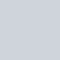# 整除與取餘數的公式

## 整除

``````=QUOTIENT(5, 2)
# 答案 = 2
``````

## 取餘數

``````=MOD(5,2)
# 答案 = 1
``````

# 實作快速轉換 「秒數」快速轉換「時、分、秒」

1. 秒/3600，「取整數」就會是小時，
2. (秒-「整小時*3600」)/60，「取整數」就會是分，
3. 而剩下的 (秒-「整小時3600」- 「整分60」) 就會是秒數。

## 需要經過變數處理的公式

``````origin_sec = 總秒數
hr = QUOTIENT(origin_sec, 3600)
min = QUOTIENT((origin_sec - hr * 3600), 60)
sec  = origin_sec - hr * 3600 - min * 60
``````

## 無變數，可以直接算的公式

``````origin_sec = 總秒數
hr = QUOTIENT(origin_sec, 3600)
min = QUOTIENT((origin_sec - QUOTIENT(origin_sec, 3600) * 3600), 60)
sec  = origin_sec - QUOTIENT(origin_sec, 3600) * 3600 -  QUOTIENT((origin_sec - QUOTIENT(origin_sec, 3600) * 3600), 60) * 60
``````

## 範例

``````A2 = 86400
hr = =QUOTIENT(A2, 3600)
min = QUOTIENT((A2 - QUOTIENT(A2, 3600) * 3600), 60)
sec = A2 - QUOTIENT(A2, 3600) * 3600 -  QUOTIENT((A2 - QUOTIENT(A2, 3600) * 3600), 60) * 60
``````

# Reference